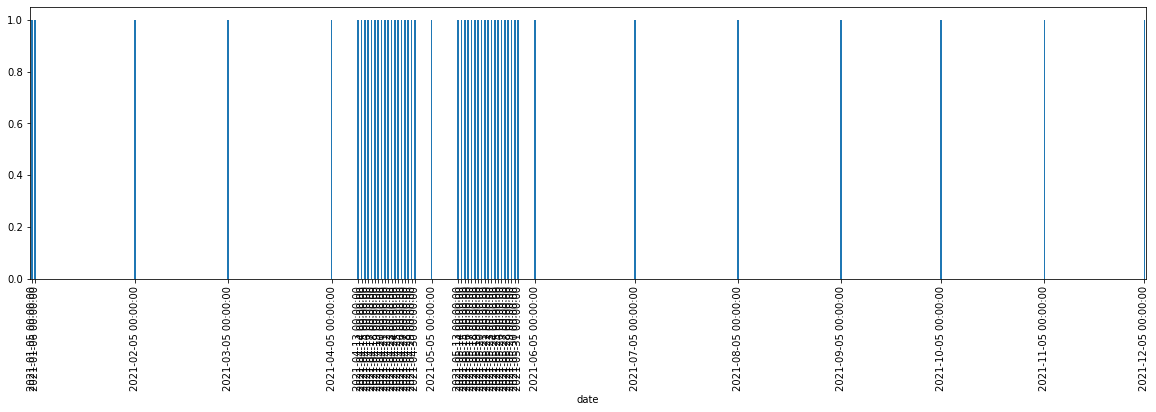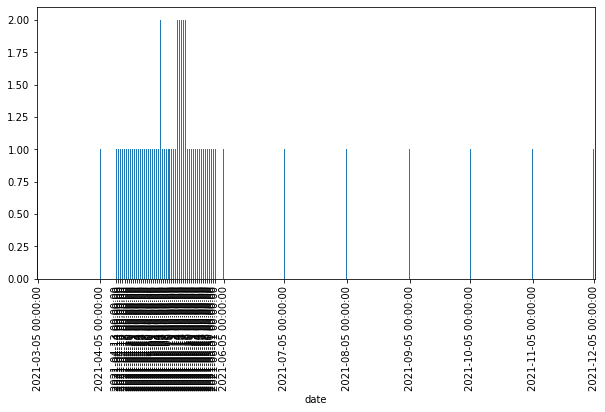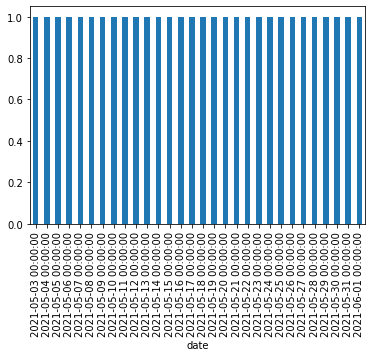In this article, we'll learn how to convert dates saved as strings in Pandas with method to_datetime. We will cover the basic usage, problematic dates and errors.

For this article we are going to generate dates with the code below:

## 1: Convert Strings to Datetime in Pandas DataFrame

First let's show how to convert a list of dates stored as strings to datetime in a DataFrame.

``````import pandas as pd

df = pd.DataFrame({'date_str':['05/18/2021', '05/19/2021', '05/20/2021']})
``````

We have a DataFrame with 1 column with several dates. The conversion to datetime column is done by:

``````df['date'] = pd.to_datetime(df['date_str'])
``````

We need to provide the column and the method will convert anything which is like a date.

Note: To check the column type, is it a string or datetime, we are going to use: `df.dtypes`. This results into:

``````date_str            object
date        datetime64[ns]
``````

## 2: Typical Errors with Pandas to_datetime

In case of errors you will get: `ParserError`. Simple example:

``````df = pd.DataFrame({'date_str':['0']})
``````

This will bring error:

ParserError: day is out of range for month: 0

or another frequent error is produced by:

``````df = pd.DataFrame({'date_str':['a']})
``````

results in:

ValueError: Given date string not likely a datetime.

Those errors are resolved by adding parameter - `errors`:

``````pd.to_datetime(df['date_str'], errors='coerce')
``````

or

``````pd.to_datetime(df['date_str'], errors='ignore')
``````

Where options are:

• errors : {'ignore', 'raise', 'coerce'}, default 'raise'
• If 'raise', then invalid parsing will raise an exception.
• If 'coerce', then invalid parsing will be set as NaT.
• If 'ignore', then invalid parsing will return the input.

## 3: Fix Pandas to_datetime produces wrong dates

Sometimes you will end with successful conversion without Python errors. Yet you may face unexpected results.

### Single format wrong dates

With the code below we are going to generate 50 dates with the same format: `%d/%m/%Y`:

``````def make_dates():
dates = []
for i in range(50, 0, -1):
day = (datetime.now() - timedelta(days=i)).date().strftime('%d/%m/%Y')
dates.append(day)
return dates
``````

Basic conversion of the those dates will produce unexpected results:

``````df['date'] = pd.to_datetime(df['date_str'])

df.groupby(['date']).date_str.count().plot(kind='bar', figsize=(20,5))
``````So `07/05/2021` is treated as `05/07/2021`. The same is for `08/05/2021`, `09/05/2021`.

To fix this date parsing problems we can use next syntax:

`pd.to_datetime(df['date_str'], format='%d/%m/%Y')`

which will produce correct datetime conversion by forcing the date format:### Two formats wrong dates

We are going to produce a list of dates for the last 30 days. First 15 will be with format: `'%m/%d/%Y'` and second half will be `'%d/%m/%Y'`:

``````from datetime import datetime, timedelta

def make_dates():
dates = []
for i in range(15, 0, -1):
day = (datetime.now() - timedelta(days=i)).date().strftime('%m/%d/%Y')
dates.append(day)
for i in range(30, 15, -1):
day = (datetime.now() - timedelta(days=i)).date().strftime('%d/%m/%Y')
dates.append(day)
return dates
``````

The expected output of the code is:

['05/18/2021',
'05/19/2021',
'05/20/2021',
'05/21/2021',
...
'15/05/2021',
'16/05/2021',
'17/05/2021']

If we try direct conversion of the DataFrame above we might get unexpected results showing the plot below:The problem above will be the result of mixed formats which is not obvious. You may need to analyze your data before conversion. In next section is the solution of this problem

## 4: Pandas to_datetime convert several formats

Let's continue from the last section and convert the same DataFrame with two and more date formats.

Below you can see how to convert string dates when the formats are clear. First we are going to parse the first format with `format='%d/%m/%Y', errors='coerce'` and then the second one will be processed plus mask:

``````df['date'] = pd.to_datetime(df['date_str'], format='%d/%m/%Y', errors='coerce')